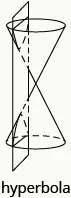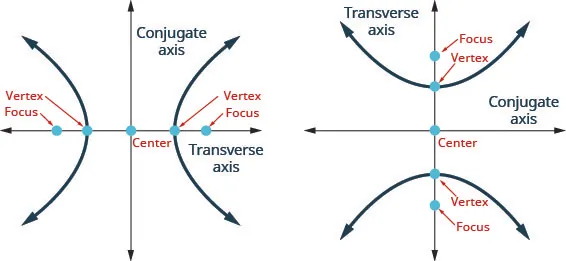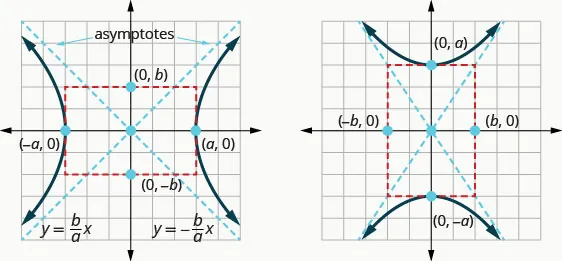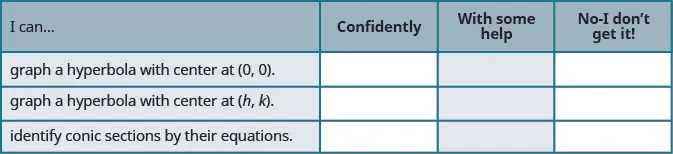Intermediate Algebra

# 11.4Hyperbolas

Intermediate Algebra11.4 Hyperbolas

### Learning Objectives

By the end of this section, you will be able to:
• Graph a hyperbola with center at $(0,0)(0,0)$
• Graph a hyperbola with center at $(h,k)(h,k)$
• Identify conic sections by their equations

### Be Prepared 11.4

Before you get started, take this readiness quiz.

1. Solve: $x2=12.x2=12.$
If you missed this problem, review Example 9.1.
2. Expand: $(x−4)2.(x−4)2.$
If you missed this problem, review Example 5.32.
3. Graph $y=−23x.y=−23x.$
If you missed this problem, review Example 3.4.

### Graph a Hyperbola with Center at (0, 0)

The last conic section we will look at is called a hyperbola. We will see that the equation of a hyperbola looks the same as the equation of an ellipse, except it is a difference rather than a sum. While the equations of an ellipse and a hyperbola are very similar, their graphs are very different.

We define a hyperbola as all points in a plane where the difference of their distances from two fixed points is constant. Each of the fixed points is called a focus of the hyperbola.

### Hyperbola

A hyperbola is all points in a plane where the difference of their distances from two fixed points is constant. Each of the fixed points is called a focus of the hyperbola.The line through the foci, is called the transverse axis. The two points where the transverse axis intersects the hyperbola are each a vertex of the hyperbola. The midpoint of the segment joining the foci is called the center of the hyperbola. The line perpendicular to the transverse axis that passes through the center is called the conjugate axis. Each piece of the graph is called a branch of the hyperbola.Again our goal is to connect the geometry of a conic with algebra. Placing the hyperbola on a rectangular coordinate system gives us that opportunity. In the figure, we placed the hyperbola so the foci $((−c,0),(c,0))((−c,0),(c,0))$ are on the x-axis and the center is the origin.The definition states the difference of the distance from the foci to a point $(x,y)(x,y)$ is constant. So $|d1−d2||d1−d2|$ is a constant that we will call $2a2a$ so $|d1−d2|=2a.|d1−d2|=2a.$ We will use the distance formula to lead us to an algebraic formula for an ellipse.

$|d1−d2|=2aUse the distance formula to findd1,d2|(x−(−c))2+(y−0)2−(x−c)2+(y−0)2|=2aEliminate the radicals.To simplify the equation of the ellipse, weletc2−a2=b2.x2a2+y2c2−a2=1So, the equation of a hyperbola centered atthe origin in standard form is:x2a2−y2b2=1|d1−d2|=2aUse the distance formula to findd1,d2|(x−(−c))2+(y−0)2−(x−c)2+(y−0)2|=2aEliminate the radicals.To simplify the equation of the ellipse, weletc2−a2=b2.x2a2+y2c2−a2=1So, the equation of a hyperbola centered atthe origin in standard form is:x2a2−y2b2=1$

To graph the hyperbola, it will be helpful to know about the intercepts. We will find the x-intercepts and y-intercepts using the formula.

$x-interceptsy-intercepts x2a2−y2b2=1x2a2−y2b2=1 Lety=0.x2a2−02b2=1Letx=0.02a2−y2b2=1 x2a2=1−y2b2=1 x2=a2y2=−b2 x=±ay=±−b2 Thex-intercepts are(a,0)and(−a,0).There are noy-intercepts.x-interceptsy-intercepts x2a2−y2b2=1x2a2−y2b2=1 Lety=0.x2a2−02b2=1Letx=0.02a2−y2b2=1 x2a2=1−y2b2=1 x2=a2y2=−b2 x=±ay=±−b2 Thex-intercepts are(a,0)and(−a,0).There are noy-intercepts.$

The a, b values in the equation also help us find the asymptotes of the hyperbola. The asymptotes are intersecting straight lines that the branches of the graph approach but never intersect as the x, y values get larger and larger.

To find the asymptotes, we sketch a rectangle whose sides intersect the x-axis at the vertices $(−a,0),(−a,0),$ $(a,0)(a,0)$ and intersect the y-axis at $(0,−b),(0,−b),$ $(0,b).(0,b).$ The lines containing the diagonals of this rectangle are the asymptotes of the hyperbola. The rectangle and asymptotes are not part of the hyperbola, but they help us graph the hyperbola.The asymptotes pass through the origin and we can evaluate their slope using the rectangle we sketched. They have equations $y=baxy=bax$ and $y=−bax.y=−bax.$

There are two equations for hyperbolas, depending whether the transverse axis is vertical or horizontal. We can tell whether the transverse axis is horizontal by looking at the equation. When the equation is in standard form, if the x2-term is positive, the transverse axis is horizontal. When the equation is in standard form, if the y2-term is positive, the transverse axis is vertical.

The second equations could be derived similarly to what we have done. We will summarize the results here.

### Standard Form of the Equation a Hyperbola with Center $( 0 , 0 ) ( 0 , 0 )$

The standard form of the equation of a hyperbola with center $(0,0),(0,0),$ is

$x2a2−y2b2=1ory2a2−x2b2=1x2a2−y2b2=1ory2a2−x2b2=1$Notice that, unlike the equation of an ellipse, the denominator of $x2x2$ is not always $a2a2$ and the denominator of $y2y2$ is not always $b2.b2.$

Notice that when the $x2x2$-term is positive, the transverse axis is on the x-axis. When the $y2y2$-term is positive, the transverse axis is on the y-axis.

Standard Forms of the Equation a Hyperbola with Center $(0,0)(0,0)$
$x2a2−y2b2=1x2a2−y2b2=1$ $y2a2−x2b2=1y2a2−x2b2=1$
Orientation Transverse axis on the x-axis.
Opens left and right
Transverse axis on the y-axis.
Opens up and down
Vertices $(−a,0),(−a,0),$ $(a,0)(a,0)$ $(0,−a),(0,−a),$ $(0,a)(0,a)$
x-intercepts $(−a,0),(−a,0),$ $(a,0)(a,0)$ none
y-intercepts none $(0,−a),(0,−a),$ $(0,a)(0,a)$
Rectangle Use $(±a,0)(±a,0)$ $(0,±b)(0,±b)$ Use $(0,±a)(0,±a)$ $(±b,0)(±b,0)$
asymptotes $y=bax,y=bax,$ $y=−baxy=−bax$ $y=abx,y=abx,$ $y=−abxy=−abx$

We will use these properties to graph hyperbolas.

### Example 11.27

#### How to Graph a Hyperbola with Center $(0,0)(0,0)$

Graph $x225−y24=1.x225−y24=1.$

### Try It 11.53

Graph $x216−y24=1.x216−y24=1.$

### Try It 11.54

Graph $x29−y216=1.x29−y216=1.$

We summarize the steps for reference.

### How To

#### Graph a hyperbola centered at $(0,0).(0,0).$

1. Step 1. Write the equation in standard form.
2. Step 2. Determine whether the transverse axis is horizontal or vertical.
3. Step 3. Find the vertices.
4. Step 4. Sketch the rectangle centered at the origin intersecting one axis at $±a±a$ and the other at $±b.±b.$
5. Step 5. Sketch the asymptotes—the lines through the diagonals of the rectangle.
6. Step 6. Draw the two branches of the hyperbola.

Sometimes the equation for a hyperbola needs to be first placed in standard form before we graph it.

### Example 11.28

Graph $4y2−16x2=64.4y2−16x2=64.$

### Try It 11.55

Graph $4y2−25x2=100.4y2−25x2=100.$

### Try It 11.56

Graph $25y2−9x2=225.25y2−9x2=225.$

### Graph a Hyperbola with Center at $(h,k)(h,k)$

Hyperbolas are not always centered at the origin. When a hyperbola is centered at $(h,k)(h,k)$ the equations changes a bit as reflected in the table.

Standard Forms of the Equation a Hyperbola with Center $(h,k)(h,k)$
$(x−h)2a2−(y−k)2b2=1(x−h)2a2−(y−k)2b2=1$ $(y−k)2a2−(x−h)2b2=1(y−k)2a2−(x−h)2b2=1$
Orientation Transverse axis is horizontal.
Opens left and right
Transverse axis is vertical.
Opens up and down
Center $(h,k)(h,k)$ $(h,k)(h,k)$
Vertices a units to the left and right of the center a units above and below the center
Rectangle Use a units left/right of center
b units above/ below the center
Use a units above/below the center
b units left/right of center

### Example 11.29

#### How to Graph a Hyperbola with Center $(h,k)(h,k)$

Graph $(x−1)29−(y−2)216=1(x−1)29−(y−2)216=1$

### Try It 11.57

Graph $(x−3)225−(y−1)29=1.(x−3)225−(y−1)29=1.$

### Try It 11.58

Graph $(x−2)24−(y−2)29=1.(x−2)24−(y−2)29=1.$

We summarize the steps for easy reference.

### How To

#### Graph a hyperbola centered at $(h,k).(h,k).$

1. Step 1. Write the equation in standard form.
2. Step 2. Determine whether the transverse axis is horizontal or vertical.
3. Step 3. Find the center and a, b.
4. Step 4. Sketch the rectangle centered at $(h,k)(h,k)$ using a, b.
5. Step 5. Sketch the asymptotes—the lines through the diagonals of the rectangle. Mark the vertices.
6. Step 6. Draw the two branches of the hyperbola.

Be careful as you identify the center. The standard equation has $x−hx−h$ and $y−ky−k$ with the center as $(h,k).(h,k).$

### Example 11.30

Graph $(y+2)29−(x+1)24=1.(y+2)29−(x+1)24=1.$

### Try It 11.59

Graph $(y+3)216−(x+2)29=1.(y+3)216−(x+2)29=1.$

### Try It 11.60

Graph $(y+2)29−(x+2)29=1.(y+2)29−(x+2)29=1.$

Again, sometimes we have to put the equation in standard form as our first step.

### Example 11.31

Write the equation in standard form and graph $4x2−9y2−24x−36y−36=0.4x2−9y2−24x−36y−36=0.$

### Try It 11.61

Write the equation in standard form and graph $9x2−16y2+18x+64y−199=0.9x2−16y2+18x+64y−199=0.$

### Try It 11.62

Write the equation in standard form and graph $16x2−25y2+96x−50y−281=0.16x2−25y2+96x−50y−281=0.$

### Identify Conic Sections by their Equations

Now that we have completed our study of the conic sections, we will take a look at the different equations and recognize some ways to identify a conic by its equation. When we are given an equation to graph, it is helpful to identify the conic so we know what next steps to take.

To identify a conic from its equation, it is easier if we put the variable terms on one side of the equation and the constants on the other.

Conic Characteristics of $x2-x2-$ and $y2-y2-$ terms Example
Parabola Either $x2x2$ OR $y2.y2.$ Only one variable is squared. $x=3y2−2y+1x=3y2−2y+1$
Circle $x2-x2-$ and $y2-y2-$ terms have the same coefficients $x2+y2=49x2+y2=49$
Ellipse $x2-x2-$ and $y2-y2-$ terms have the same sign, different coefficients $4x2+25y2=1004x2+25y2=100$
Hyperbola $x2-x2-$ and $y2-y2-$ terms have different signs, different coefficients $25y2−4x2=10025y2−4x2=100$

### Example 11.32

Identify the graph of each equation as a circle, parabola, ellipse, or hyperbola.

$9x2+4y2+56y+160=09x2+4y2+56y+160=0$ $9x2−16y2+18x+64y−199=09x2−16y2+18x+64y−199=0$ $x2+y2−6x−8y=0x2+y2−6x−8y=0$ $y=−2x2−4x−5y=−2x2−4x−5$

### Try It 11.63

Identify the graph of each equation as a circle, parabola, ellipse, or hyperbola.

$x2+y2−8x−6y=0x2+y2−8x−6y=0$ $4x2+25y2=1004x2+25y2=100$ $y=6x2+2x−1y=6x2+2x−1$ $16y2−9x2=14416y2−9x2=144$

### Try It 11.64

Identify the graph of each equation as a circle, parabola, ellipse, or hyperbola.

$16x2+9y2=14416x2+9y2=144$ $y=2x2+4x+6y=2x2+4x+6$ $x2+y2+2x+6y+9=0x2+y2+2x+6y+9=0$ $4x2−16y2=644x2−16y2=64$

### Media

Access these online resources for additional instructions and practice with hyperbolas.

### Section 11.4 Exercises

#### Practice Makes Perfect

Graph a Hyperbola with Center at $(0,0)(0,0)$

In the following exercises, graph.

147.

$x 2 9 − y 2 4 = 1 x 2 9 − y 2 4 = 1$

148.

$x 2 25 − y 2 9 = 1 x 2 25 − y 2 9 = 1$

149.

$x 2 16 − y 2 25 = 1 x 2 16 − y 2 25 = 1$

150.

$x 2 9 − y 2 36 = 1 x 2 9 − y 2 36 = 1$

151.

$y 2 25 − x 2 4 = 1 y 2 25 − x 2 4 = 1$

152.

$y 2 36 − x 2 16 = 1 y 2 36 − x 2 16 = 1$

153.

$16 y 2 − 9 x 2 = 144 16 y 2 − 9 x 2 = 144$

154.

$25 y 2 − 9 x 2 = 225 25 y 2 − 9 x 2 = 225$

155.

$4 y 2 − 9 x 2 = 36 4 y 2 − 9 x 2 = 36$

156.

$16 y 2 − 25 x 2 = 400 16 y 2 − 25 x 2 = 400$

157.

$4 x 2 − 16 y 2 = 64 4 x 2 − 16 y 2 = 64$

158.

$9 x 2 − 4 y 2 = 36 9 x 2 − 4 y 2 = 36$

Graph a Hyperbola with Center at $(h,k)(h,k)$

In the following exercises, graph.

159.

$( x − 1 ) 2 16 − ( y − 3 ) 2 4 = 1 ( x − 1 ) 2 16 − ( y − 3 ) 2 4 = 1$

160.

$( x − 2 ) 2 4 − ( y − 3 ) 2 16 = 1 ( x − 2 ) 2 4 − ( y − 3 ) 2 16 = 1$

161.

$( y − 4 ) 2 9 − ( x − 2 ) 2 25 = 1 ( y − 4 ) 2 9 − ( x − 2 ) 2 25 = 1$

162.

$( y − 1 ) 2 25 − ( x − 4 ) 2 16 = 1 ( y − 1 ) 2 25 − ( x − 4 ) 2 16 = 1$

163.

$( y + 4 ) 2 25 − ( x + 1 ) 2 36 = 1 ( y + 4 ) 2 25 − ( x + 1 ) 2 36 = 1$

164.

$( y + 1 ) 2 16 − ( x + 1 ) 2 4 = 1 ( y + 1 ) 2 16 − ( x + 1 ) 2 4 = 1$

165.

$( y − 4 ) 2 16 − ( x + 1 ) 2 25 = 1 ( y − 4 ) 2 16 − ( x + 1 ) 2 25 = 1$

166.

$( y + 3 ) 2 16 − ( x − 3 ) 2 36 = 1 ( y + 3 ) 2 16 − ( x − 3 ) 2 36 = 1$

167.

$( x − 3 ) 2 25 − ( y + 2 ) 2 9 = 1 ( x − 3 ) 2 25 − ( y + 2 ) 2 9 = 1$

168.

$( x + 2 ) 2 4 − ( y − 1 ) 2 9 = 1 ( x + 2 ) 2 4 − ( y − 1 ) 2 9 = 1$

In the following exercises, write the equation in standard form and graph.

169.

$9 x 2 − 4 y 2 − 18 x + 8 y − 31 = 0 9 x 2 − 4 y 2 − 18 x + 8 y − 31 = 0$

170.

$16 x 2 − 4 y 2 + 64 x − 24 y − 36 = 0 16 x 2 − 4 y 2 + 64 x − 24 y − 36 = 0$

171.

$y 2 − x 2 − 4 y + 2 x − 6 = 0 y 2 − x 2 − 4 y + 2 x − 6 = 0$

172.

$4 y 2 − 16 x 2 − 24 y + 96 x − 172 = 0 4 y 2 − 16 x 2 − 24 y + 96 x − 172 = 0$

173.

$9 y 2 − x 2 + 18 y − 4 x − 4 = 0 9 y 2 − x 2 + 18 y − 4 x − 4 = 0$

Identify the Graph of each Equation as a Circle, Parabola, Ellipse, or Hyperbola

In the following exercises, identify the type of graph.

174.

$x=−y2−2y+3x=−y2−2y+3$ $9y2−x2+18y−4x−4=09y2−x2+18y−4x−4=0$ $9x2+25y2=2259x2+25y2=225$ $x2+y2−4x+10y−7=0x2+y2−4x+10y−7=0$

175.

$x=−2y2−12y−16x=−2y2−12y−16$ $x2+y2=9x2+y2=9$ $16x2−4y2+64x−24y−36=016x2−4y2+64x−24y−36=0$ $16x2+36y2=57616x2+36y2=576$

Mixed Practice

In the following exercises, graph each equation.

176.

$( y − 3 ) 2 9 − ( x + 2 ) 2 16 = 1 ( y − 3 ) 2 9 − ( x + 2 ) 2 16 = 1$

177.

$x 2 + y 2 − 4 x + 10 y − 7 = 0 x 2 + y 2 − 4 x + 10 y − 7 = 0$

178.

$y = ( x − 1 ) 2 + 2 y = ( x − 1 ) 2 + 2$

179.

$x 2 9 + y 2 25 = 1 x 2 9 + y 2 25 = 1$

180.

$( x + 2 ) 2 + ( y − 5 ) 2 = 4 ( x + 2 ) 2 + ( y − 5 ) 2 = 4$

181.

$9 x 2 − 4 y 2 + 54 x + 8 y + 41 = 0 9 x 2 − 4 y 2 + 54 x + 8 y + 41 = 0$

182.

$x = − y 2 − 2 y + 3 x = − y 2 − 2 y + 3$

183.

$16 x 2 + 9 y 2 = 144 16 x 2 + 9 y 2 = 144$

#### Writing Exercises

184.

In your own words, define a hyperbola and write the equation of a hyperbola centered at the origin in standard form. Draw a sketch of the hyperbola labeling the center, vertices, and asymptotes.

185.

Explain in your own words how to create and use the rectangle that helps graph a hyperbola.

186.

Compare and contrast the graphs of the equations $x24−y29=1x24−y29=1$ and $y29−x24=1.y29−x24=1.$

187.

Explain in your own words, how to distinguish the equation of an ellipse with the equation of a hyperbola.

#### Self Check

After completing the exercises, use this checklist to evaluate your mastery of the objectives of this section.On a scale of 1-10, how would you rate your mastery of this section in light of your responses on the checklist? How can you improve this?

Order a print copy

As an Amazon Associate we earn from qualifying purchases.